# 2 Derivatives 2 1 Derivatives and Rates of

• Slides: 19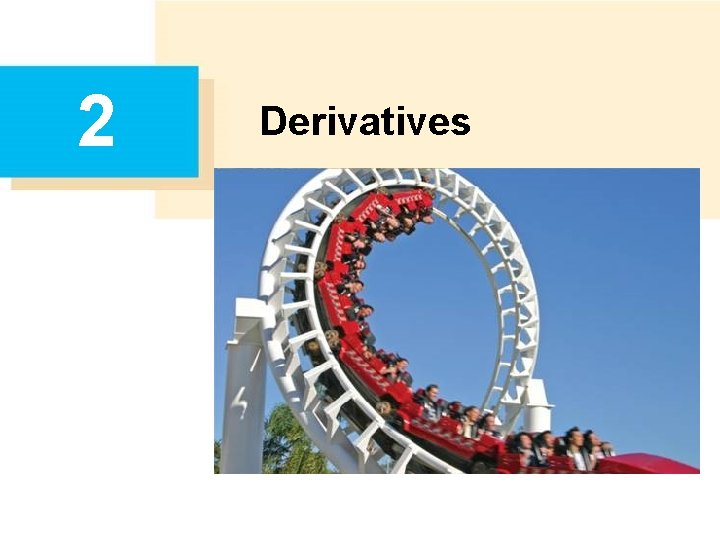2 Derivatives2. 1 Derivatives and Rates of Change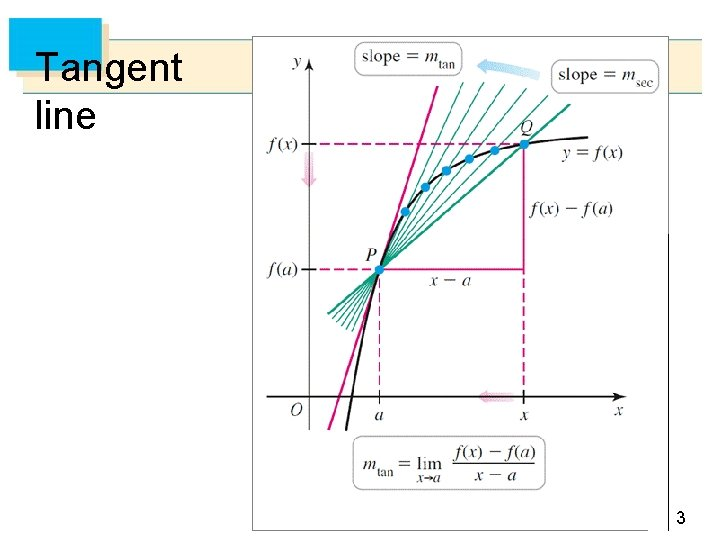Tangent line 3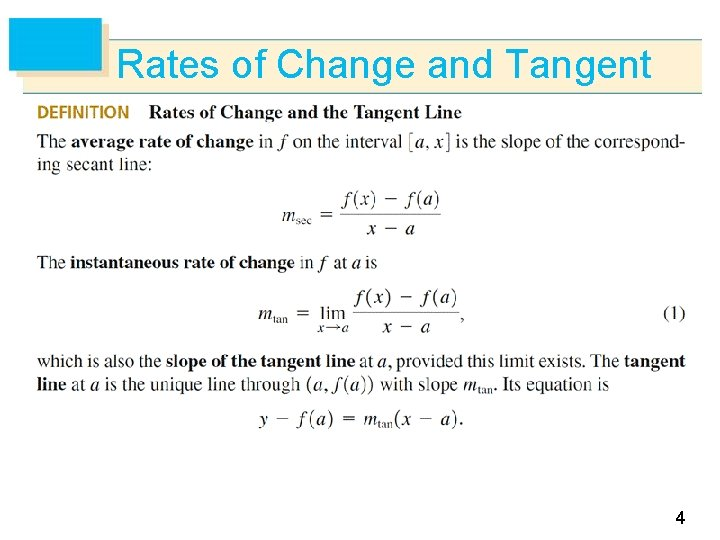Rates of Change and Tangent 4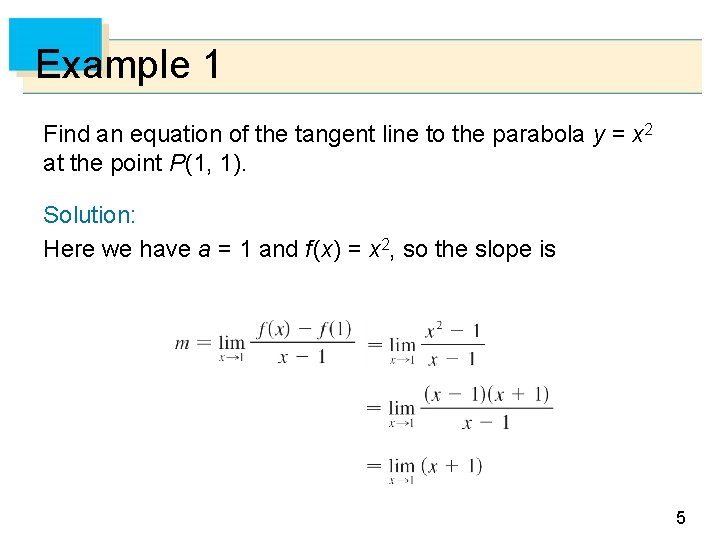Example 1 Find an equation of the tangent line to the parabola y = x 2 at the point P(1, 1). Solution: Here we have a = 1 and f (x) = x 2, so the slope is 5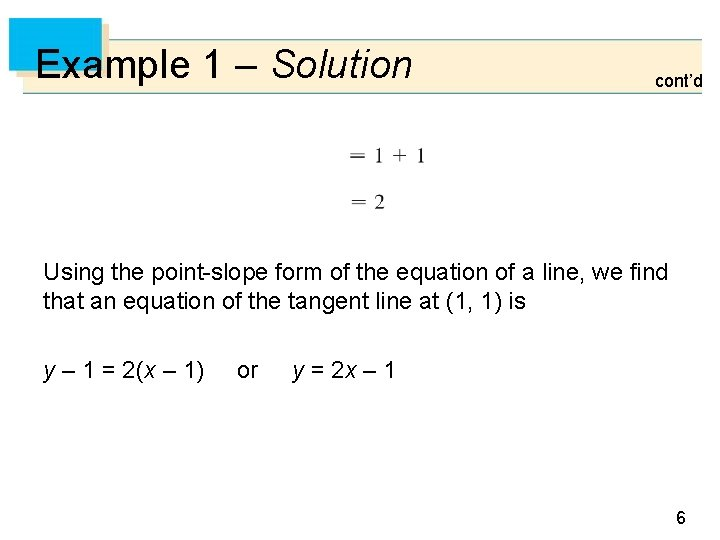Example 1 – Solution cont’d Using the point-slope form of the equation of a line, we find that an equation of the tangent line at (1, 1) is y – 1 = 2(x – 1) or y = 2 x – 1 6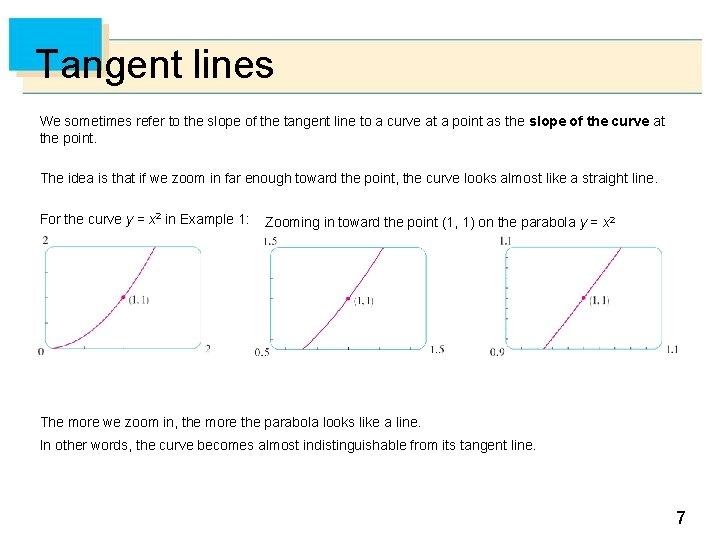Tangent lines We sometimes refer to the slope of the tangent line to a curve at a point as the slope of the curve at the point. The idea is that if we zoom in far enough toward the point, the curve looks almost like a straight line. For the curve y = x 2 in Example 1: Zooming in toward the point (1, 1) on the parabola y = x 2 The more we zoom in, the more the parabola looks like a line. In other words, the curve becomes almost indistinguishable from its tangent line. 7Alternative notation: (more useful) Let x = a+h in the previous notation 8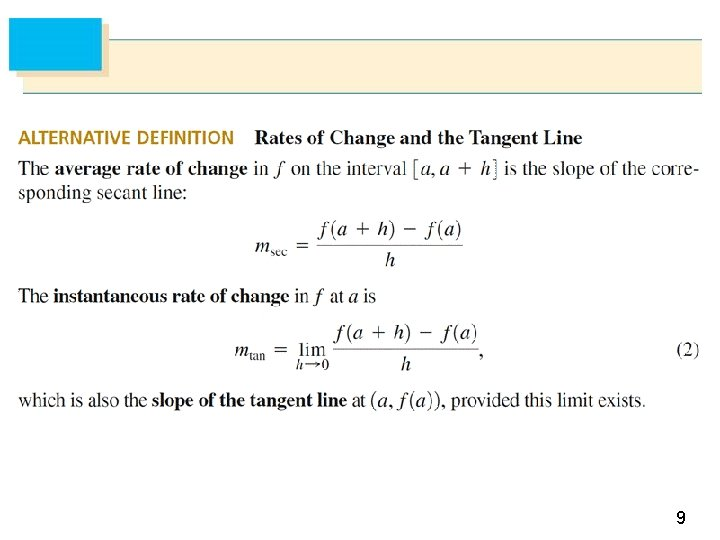9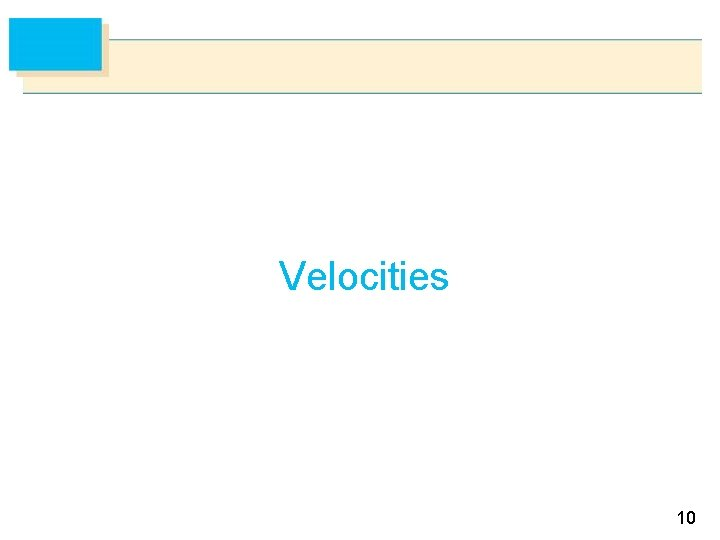Velocities 10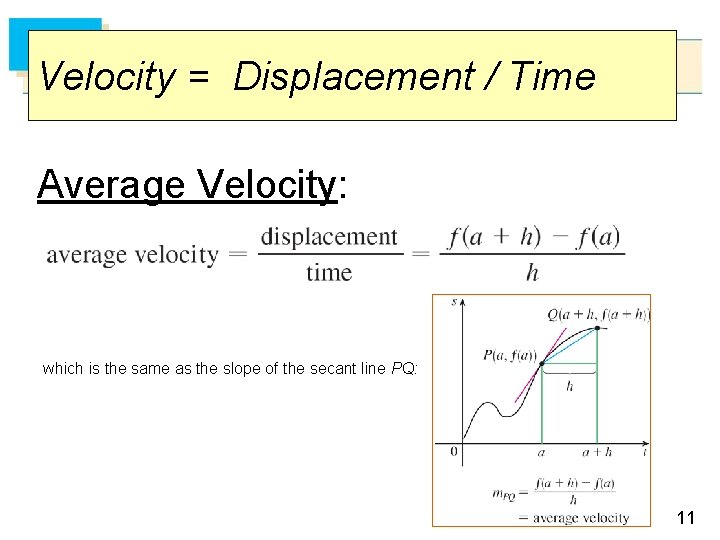Velocity = Displacement / Time Average Velocity: which is the same as the slope of the secant line PQ: 11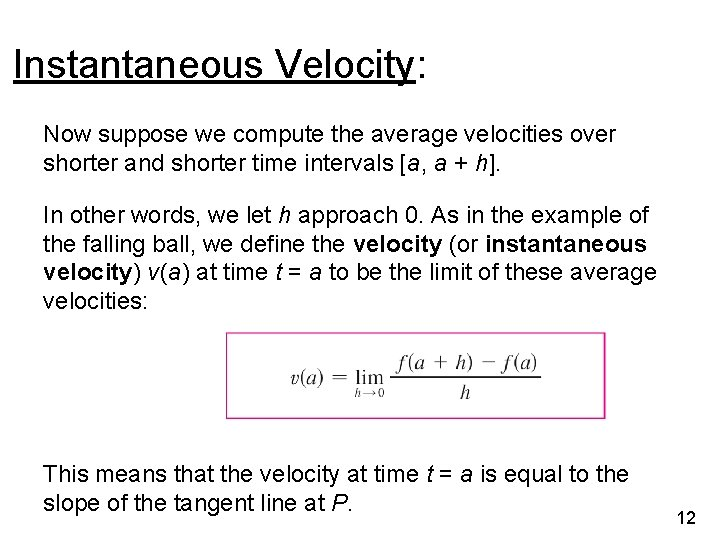Instantaneous Velocity: Now suppose we compute the average velocities over shorter and shorter time intervals [a, a + h]. In other words, we let h approach 0. As in the example of the falling ball, we define the velocity (or instantaneous velocity) v (a) at time t = a to be the limit of these average velocities: This means that the velocity at time t = a is equal to the slope of the tangent line at P. 12Example Suppose that a ball is dropped from the upper observation deck of the CN Tower, 450 m above the ground. The height of the ball is s = f (t) = 4. 9 t 2 at any given time t during its fall. (Note: at t=0 the ball is at the top of the tower). (a) What is the velocity of the ball after 5 seconds? (*first find it at any time “a”) (b) How fast is the ball traveling when it hits the ground? Solution: We will need to find the velocity both when t = 5 and when the ball hits the ground, so it’s efficient to start by finding the velocity at a general time t = a. 13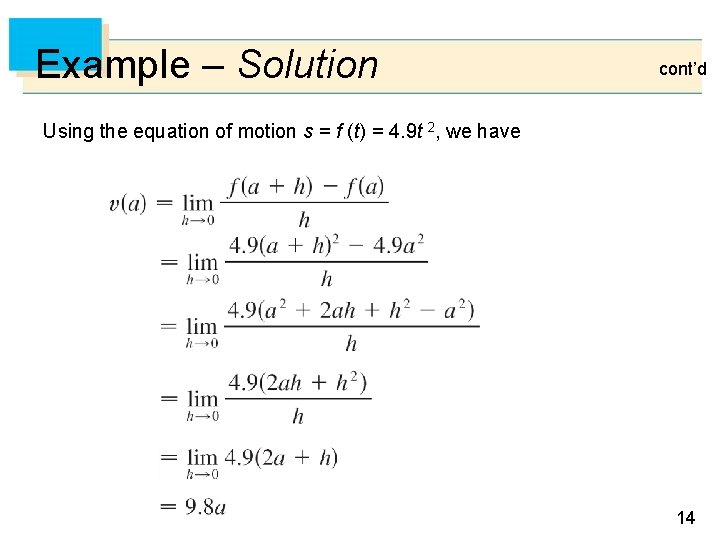Example – Solution cont’d Using the equation of motion s = f (t) = 4. 9 t 2, we have 14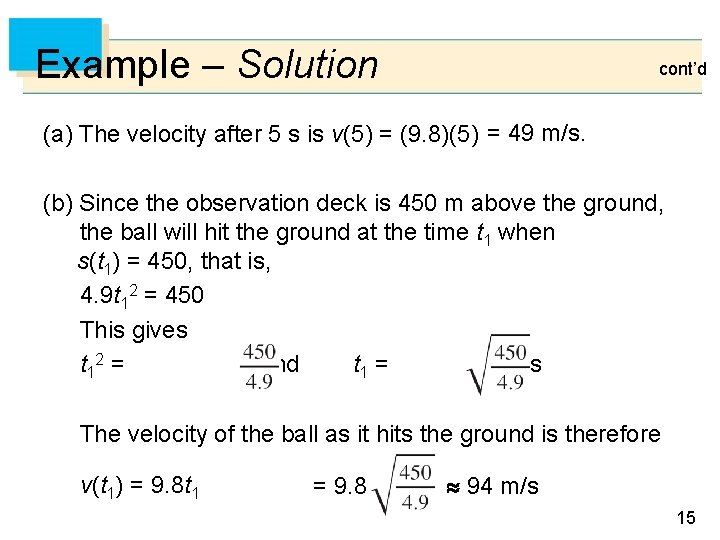Example – Solution cont’d (a) The velocity after 5 s is v(5) = (9. 8)(5) = 49 m/s. (b) Since the observation deck is 450 m above the ground, the ball will hit the ground at the time t 1 when s(t 1) = 450, that is, 4. 9 t 12 = 450 This gives t 12 = and t 1 = 9. 6 s The velocity of the ball as it hits the ground is therefore v(t 1) = 9. 8 t 1 = 9. 8 94 m/s 15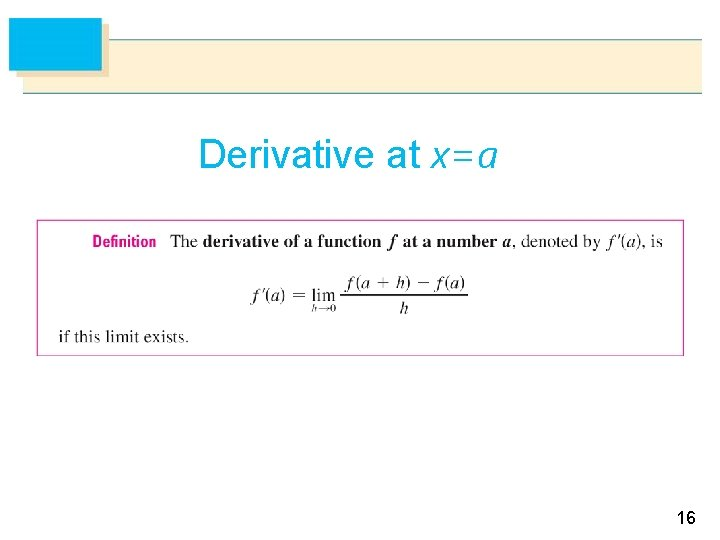Derivative at x=a 16Example Find the derivative of the function f (x) = x 2 – 8 x + 9 at the number x=a. Solution: From the definition we have 17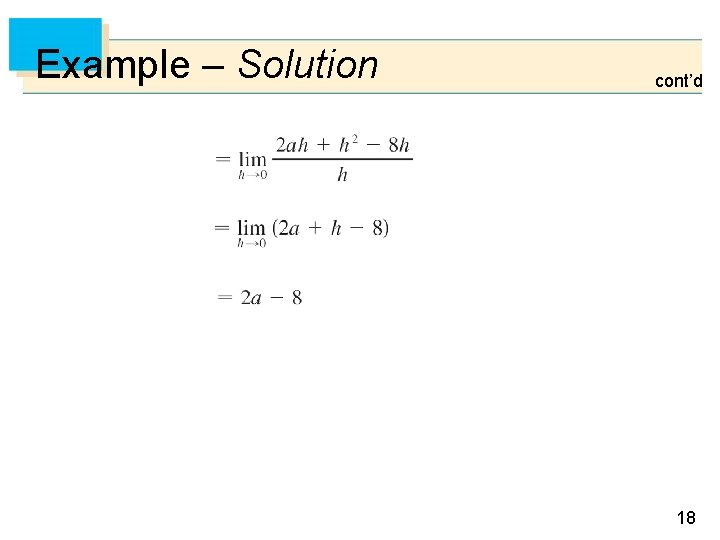Example – Solution cont’d 18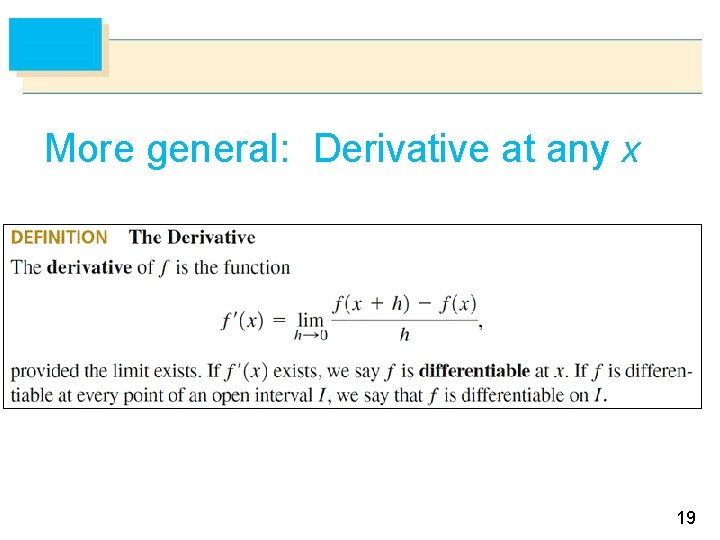More general: Derivative at any x 19